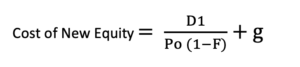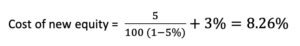# Cost of New Equity

Cost of New Equity (also known as floatation cost) is the cost associate with issuing new stock to the capital market in order to raise more funds. Floatation cost will increase the cost of new equity, as a result, it will be higher than the existing equity in the same company. It includes other costs such as underwriting fees, registration, legal fees, and other costs. These fees will impact the amount of capital to be raised.

These costs mostly the percentage of share price, so we will calculate by applying the percentage below formula.

## Cost of New Equity Formula

The cost of new equity will be calculated by using the dividend growth model:D1: Dividend at the end of next year

P0 : The issued share price

F: the ratio of the flotation cost

g: dividend growth rate

## Cost of New Equity Example

The company decided to issue \$ 500 million of new common stocks to the market. They are issued at \$ 100 per share and the broker charge fee 5% over the share price. Base on historical data, the annual dividend expected to be \$ 5 per share and it will grow at 3% rate. Please calculate the cost of new equity.Without flotation cost, the cost of equity is only 8%, so the 0.26% is the impact of the additional cost.